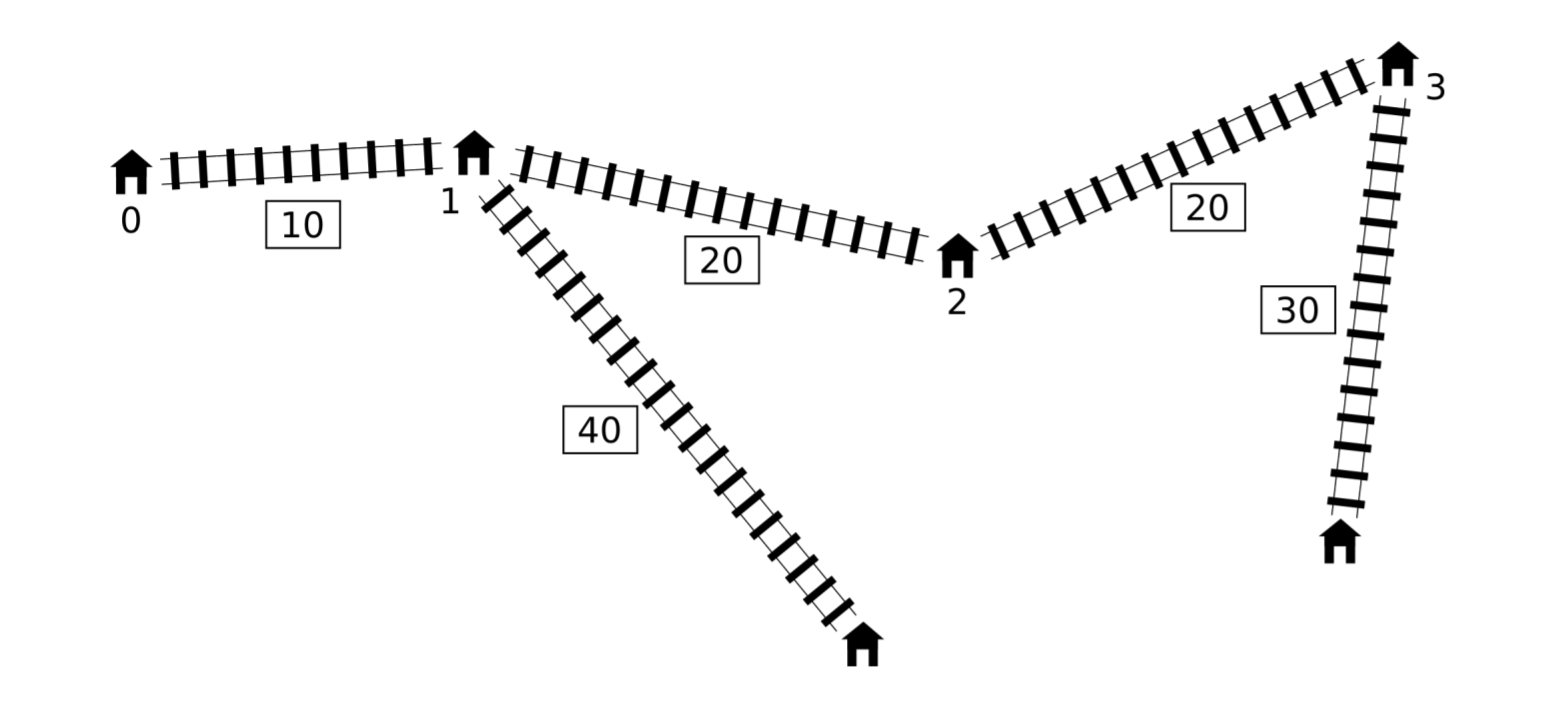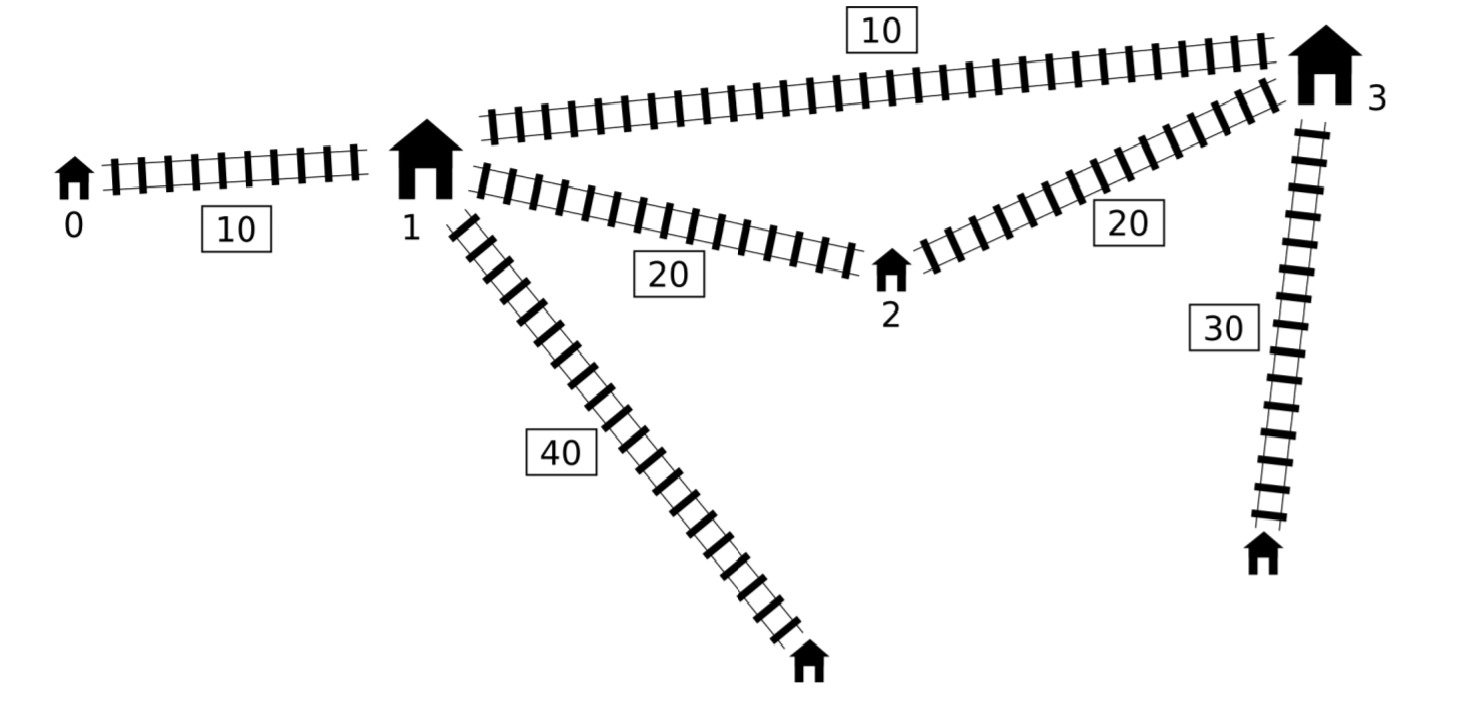## Shortcut

Pavel has a toy railway. It is very simple. There is a main line consisting of n stations. These stations are numbered from 0 to n − 1 in order along the line. The distance between the stations i and i + 1 is li centimeters (0 ≤ i < n − 1).

Apart from the main line there may be some secondary lines. Each secondary line is a railway line between a station on the main line and a new station that does not lie on the main line. (These new stations are not numbered.) At most one secondary line can start in each station of the main line. The length of the secondary line starting at station i is di centimeters. We use di = 0 to denote that there is no secondary line starting at station i.Pavel is now planning to build one shortcut: an express line between two different (possibly neighbouring) stations of the main line. The express line will have a length of exactly c centimeters, regardless of which two stations it will connect.

Each segment of the railway, including the new express line, can be used in both directions. The distance between two stations is the smallest length of a route that goes from one station to the other along the railways. The diameter of the whole railway network is the maximum distance among all pairs of stations. In other words, this is the smallest number t, such that the distance between every pair of stations is at most t.

Pavel wants to build the express line in such a way that the diameter of the resulting network is minimized.

You should implement one operation `find_shortcut`:

• `find_shortcut(n, l[], d[], c)`
• `n`: number of stations on the main line,
• `l`: distances between stations on the main line (array of length n − 1),
• `d`: lengths of secondary lines (array of length n),
• `c`: length of the new express line.
• your program should output the smallest possible diameter of the railway network after adding the express line.

### Input Format

The input will be given in the following format:

• Line 1: space-separated integers n and c
• Line 2: space-separated integers l0, …, ln − 2
• Line 3: space-separated integers d0, …, dn − 1

### Output Format

Your output should be in the following format:

• Line 1: a single integer denoting the smallest possible diameter

```4 10
10 20 20
0 40 0 30
```

`80`

### Explanation 1

The optimal solution is to build the express line between stations 1 and 3, as shown below.The diameter of the new railway network is 80 centimeters.

### Sample Input 2

```9 30
10 10 10 10 10 10 10 10
20 0 30 0 0 40 0 40 0
```

`110`

### Explanation 2

The optimal solution is to connect stations 2 and 7, in which case the diameter is 110.

```4 1
2 2 2
1 10 10 1
```

`21`

### Explanation 3

The optimal solution is to connect stations 1 and 2, reducing the diameter to 21.

```3 3
1 1
1 1 1
```

`4`

### Explanation 4

Connecting any two stations with the express line of length 3 does not improve the initial diameter of the railway network which is 4.

In all subtasks: 2 ≤ n ≤ 1 000 000, 1 ≤ li ≤ 109, 0 ≤ di ≤ 109, 1 ≤ c ≤ 109.

1. (9% of points) 2 ≤ n ≤ 10
2. (14% of points) 2 ≤ n ≤ 100
3. (8% of points) 2 ≤ n ≤ 250
4. (7% of points) 2 ≤ n ≤ 500
5. (33% of points) 2 ≤ n ≤ 3000
6. (22% of points) 2 ≤ n ≤ 100 000
7. (4% of points) 2 ≤ n ≤ 300 000
8. (3% of points) 2 ≤ n ≤ 1 000 000

Point Value: 35 (partial)
Time Limit: 3.00s
Memory Limit: 2048M